# AP Board 9th Class Maths Solutions Chapter 4 Lines and Angles Ex 4.4

AP State Syllabus AP Board 9th Class Maths Solutions Chapter 4 Lines and Angles Ex 4.4 Textbook Questions and Answers.

## AP State Syllabus 9th Class Maths Solutions 4th Lesson Lines and Angles Exercise 4.4

Question 1.
In the given triangles, find out ∠x, ∠y and ∠z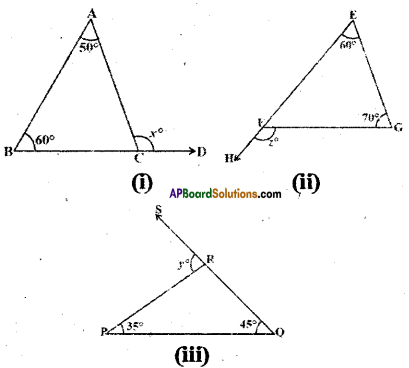Solution:
In fig(i)
x° = 50° + 60°
(∵ exterior angle is equal to sum of the opposite interior angles)
∴ x= 110°

In fig (ii)
z° = 60° + 70°
(∵ exterior angle is equal to sum of the opposite interior angles)
∴ z = 130°

In fig (iii)
y° = 35° + 45° = 80°
(∵ exterior angle is equal to sum of the opposite interior angles)Question 2.
In the given figure AS // BT; ∠4 = ∠5, $$\overline{\mathbf{S B}}$$ bisects ∠AST. Find the measure of ∠1.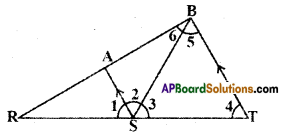Solution:
Given AS // BT
∠4 = ∠5 and SB bisects ∠AST.
∴ By problem
∠2 = ∠3 …………..(1)
For the lines AS // BT
∠2 = ∠5 ( ∵alternate interior angles)
∴ In ΔBST
∠3 = ∠5 = ∠4
Hence ΔBST is equilateral triangle and each of its angle is equal to 60°.
∴∠3 = ∠2 = 60° [by eq. (1)]
Now ∠1 + ∠2 + ∠3 = 180°
∠1 + 60° + 60° = 180°
[ ∵ angles at a point on a line]
∴∠1 = 180° – 120° = 60°

Question 3.
In the given figure AB // CD; BC // DE then find the values of x and y.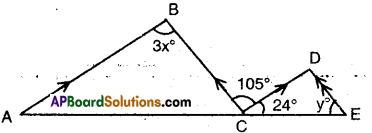Solution:
Given that AB // CD and BC // DE.
∴ 3x = 105° (∵ alternate interior angles for AB // CD)
x = $$\frac { 105° }{ 3 }$$ = 35°
Also BC // DE
∴∠D = 105°
(∵ alternate interior angles)
Now in ΔCDE
24° + 105° + y = 180°
(∵ angle sum property)
∴ y = 180° – 129° = 51°Question 4.
In the given figure BE ⊥ DA and CD ⊥ DA then prove that m∠1 = m∠3.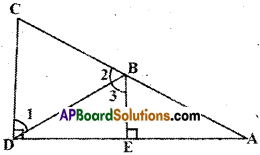Solution:
Given that CD ⊥ DA and BE ⊥ DA.
⇒ Two lines CD and BE are perpendicular to the same line DA.
⇒ CD // BE (or)
∠D =∠E ⇒ CD // BE
(∵ corresponding angles for CD and BE and DA are transversal)
Now m∠1 = m∠3
(∵alternate interior angles for the lines CD // BE ; DB are transversal)
Hence proved.

Question 5.
Find the values of x, y for which lines AD and BC become parallel.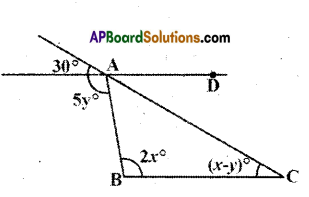Solution:
For the lines AD and BC to be parallel x – y = 30° (corresponding angles) ……… (1)
2x = 5y ………….(2)
(∵ alternate interior angles)
Solving (1) & (2)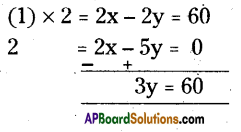y = $$\frac{60}{3}$$ = 20°
Substituting y = 20° in eq. (1)
x – 20° = 30°
⇒ x = 50°
∴ x = 50° and y = 20°Question 6.
Find the values of x and y in the figure.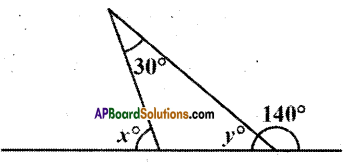Solution:
From the figure y + 140° = 180°
(∵ linear pair of angles)
∴ y = 180° – 140° = 40°
And x° = 30° + y°
(∵ exterior angle = sum of the opposite interior angles)
x° = 30° + 40° = 70°

Question 7.
In the given figure segments shown by arrow heads are parallel. Find the values of x and y.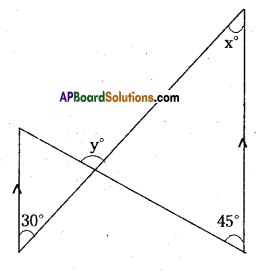Solution:
From the figure
x° = 30° (∵ alternate interior angles)
y° = 45° + x° (∵ exterior angles of a triangle = sum of opp. interior angles)
y = 45° + 30° – 75°

Question 8.
In the given figure sides QP and RQ of ∠PQR are produced to points S and T respectively. If ∠SPR = 135° and ∠PQT = 110°, find ∠PRQ.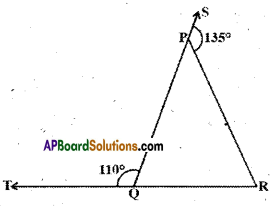Solution:
Given that ∠SPR = 135° and ∠PQT =110°
From the figure
∠SPR + ∠RPQ = 180°
∠PQT + ∠PQR = 180°
[∵ linear pair of angles]
⇒ ∠RPQ = 180° – ∠SPR
= 180° – 135° = 45°
⇒ ∠PQR = 180° – ∠PQT
= 180°-110° = 70°
Now in APQR
∠RPQ + ∠PQR + ∠PRQ = 180°
[∵ angle sum property]
∴ 45° + ’70° + ∠PRQ = 180°
∴ ∠PRQ = 180°-115° = 65°Question 9.
In the given figure ∠X = 62° ; ∠XYZ = 54°. In ΔXYZ. If YO and ZO are the bisectors of ∠XYZ and ∠XZY respec-tively find ∠OZY and ∠YOZ.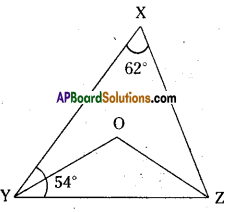Solution:
Given that ∠X = 62° and ∠Y = 54°
YO arid ZO are bisectors of ∠Y and ∠Z.
In ΔXYZ
∠X + ∠XYZ + ∠XZY = 180° .
62° + 54° + ∠XZY = 180°
=> ∠XZY = 180°- 116° = 64°
Also in Δ𝜏OYZ
∠OYZ = 1/2 ∠XYZ = 1/2 x 54° = 27°
(∵ YO is bisector of ∠XYZ)
∠OZY = 1/2 ∠XZY = 1/2 x 64° = 32
(∵ OZ is bisector of ∠XYZ)
And ∠OYZ + ∠OZY + ∠YOZ = 180°
(∵ angle sum property, ΔOYZ)
⇒ 27 + 32° + ∠YOZ = 180°
⇒ ∠YOZ = 180° – 59° = 121°

Question 10.
In the given figure if AB // DE, ∠BAC = 35° and ∠CDE = 53°, find ∠DCE.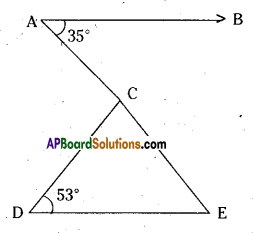Solution:
Given that AB // DE, ∠CDE = 53°;
∠BAC = 35°
Now ∠E = 35°
( ∵ alternate interior angles)
Now in ∆CDE
∠C + ∠D + ∠E = 180°
(∵angle sum property, ACDE)
∴ ∠DCE + 53° + 35° = 180°
⇒ ∠DCE = 180° – 88° = 92°Question 11.
In the given figure if line segments PQ and RS intersect at point T, such that∠PRT = 40°, ∠RPT = 95° and ∠TSQ = 75°, find ∠SQT.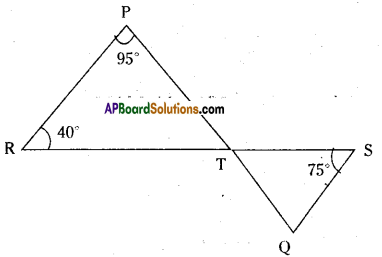Solution:
Given that ∠PRT = 40°; ∠RPT = 95°;
∠TSQ = 75°
In ∆PRT ∠P + ∠R + ∠PTR = 180°
(∵angle sum property)
95° + 40° + ∠PTR = 180°
⇒ ∠PTR = 180° – 135° = 45°
Now ∠PTR = ∠STQ
(∵ vertically opposite angles)
In ΔSTQ ∠S + ∠Q + ∠STQ = 180°
(∵ angle sum property)
75° + ∠SQT + 45° = 180°
∴ ∠SQT = 180° – 120° = 60°

Question 12.
In the given figure, ABC is a triangle in which ∠B = 50° and ∠C = 70°. Sides AB and AC are produced. If ∠ is the measure of angle between the bisec¬tors of the exterior angles so formed, then find ‘z’.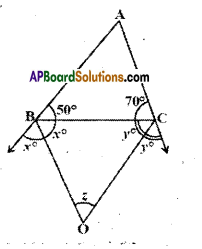Solution:
Given that ∠B = 50°; ∠C = 70°
Angle between bisectors of exterior angles B and C is ∠.
From the figure
50° + 2x = 180°
70° + 2y = 180°
(∵ linear pair of angles)
∴ 2x= 180°-50°
2x= 130°
x = $$\frac{130}{2}$$
= 65°

2y= 180°-70°
2y= 110°
x = $$\frac{110°}{2}$$
= 55°

Now in ΔBOC
x + y + ∠ = 180° (∵ angle sum property)
65° + 55° + ∠ = 180°
z = 180° -120° = 60°Question 13.
In the given figure if PQ ⊥ PS; PQ // SR, ∠SQR = 28° and ∠QRT = 65°, then find the values of x and y.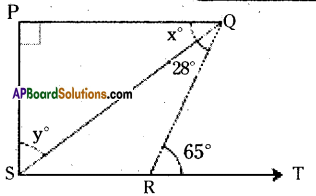Solution:
Given that PQ ⊥ PS ; PQ // SR
∠SQR = 28°, ∠QRT = 65°
From the figure
∠QSR = x° (∵ alt. int. angles for the lines PQ // SR)
Also 65° = x + 28° (∵ ext. angles = sum of the opp. interior angles)
∴ x° = 65° – 28° = 37°
And x° + y° = 90°
[ ∵ PQ ⊥ PS and PQ // SR. ⇒ ∠P = ∠S]
37° + y = 90°
∴ y = 90° – 37° = 53°

Question 14.
In the given figure ΔABC side AC has been produced to D. ∠BCD = 125° and ∠A: ∠B = 2:3, find the measure of ∠A and ∠B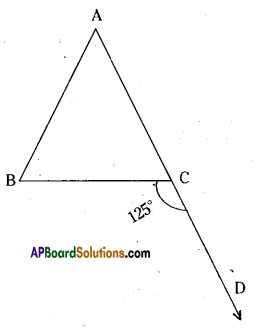Solution:
Given that ∠BCD = 125°
∠A : ∠B = 2 : 3
Sum of the terms of the ratio
∠A : ∠B = 2 + 3 = 5
We know that ∠A + ∠B = ∠BCD
(∵ exterior angles of triangle is equal to sum of its opp. interior angles)
∴ ∠A = $$\frac{2}{5}$$ x 125° = 50°
∠B = $$\frac{3}{5}$$ x 125° = 75°

Question 15.
In the given figure, it is given that, BC // DE, ∠BAC = 35° and ∠BCE = 102°. Find the measure of 0 ∠BCA i0 ∠ADE and iii) ∠CED.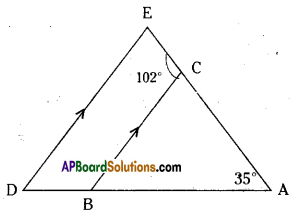Solution:
Given that BC // DE ; ∠BAC = 35°;
∠BCE = 102°

i) From the figure
102° + ∠BCA = 180°
(∵ linear pair of angles)
∴ ∠BCA = 180° – 102° = 78°ii) ∠ADE + ∠CBD = 180°
(∵ interior angles on the same side of the transversal)
∠ADE + (78° + 35°) = 180°
(∵ ∠CBD = ∠BAC + ∠BCA)
∴ ∠ADE = 180° – 113° = 67°

iii) From the figure .
∠CED = ∠BCA = 78°
(∵ corresponding angles)

Question 16.
In the given figure, it is given that AB = AC; ∠BAC = 36°; ∠ADB = 45° and ∠AEC = 40°. Find i) ∠ABC
i) ∠ACB iii) ∠DAB iv) ∠EAC.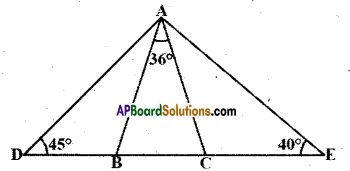Solution:
Given that AB = AC; ∠BAC = 36°,
∠ADB = 45°, ∠AEC = 40°
(i) & (ii)
In ∆ABC ; AB = AC
⇒ ∠ABC = ∠ ACB
And 36° + ∠ABC + ∠ACB = 180°
(∵ angle sum property)
∴ ∠ABC = $$\frac{180^{\circ}-36^{\circ}}{2}=\frac{144^{\circ}}{2}=72^{\circ}$$
∠ACB = 72°

iii) From the figure
∠ABD + ∠ABC = 180°
∠ABD = 180° – 72° = 1086
In ΔABD
∠DAB + ∠ABD + ∠D = 180°
∠DAB + 108° + 45° = 180°
∠DAB = 180° – 153° = 27°∠D + ∠A + ∠E = 180°
45° + ∠A + 40° = 180°
⇒ ∠A = 180° -85° = 95°
But ∠A = ∠DAB + 36° + ∠EAC
95° = 27°, + 36° + ∠EAC
∴ ∠EAC = 95° – 63° = 32°

Question 17.
Using information given in the figure, calculate the values of x and y.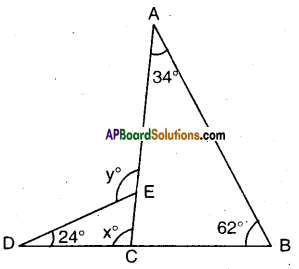Solution:
From the figure In ∆ACB
34° + 62° + ∠ACB = 180°
(∵ angle sum property)
∴ ∠ACB = 180° – 96° = 84° .
And x + ∠ACB = 180°
(∵ linear pair of angles) .
∴ x + 84° = 180°
x = 180°-84° = 96°
(OR)
x = 34° + 62° = 96°
( ∵ x is exterior angle, ∆ABC)
y = 24° + x°
= 24° + 96° = 120°
(∵ y is exterior angle, ∆DCE)